Engineering ToolBox - Resources, Tools and Basic Information for Engineering and Design of Technical Applications!

# Levers

## Use levers to magnify forces.

A lever is a mechanism that can be used to exert a large force over a small distance at one end of the lever by exerting a small force over a greater distance at the other end.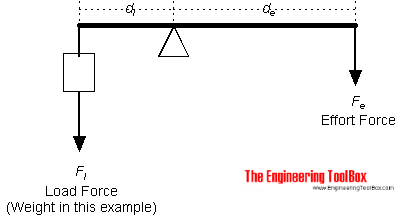The moment action on both sides of the lever is equal and can be expressed as

Fe de = Fl dl                             (1)

where

Fe = effort force (N, lb)

Fl = load force (N, lb) (note that weight is a force)

dl = distance from load force to fulcrum (m, ft)

de = distance from effort force to fulcrum (m, ft)

The effort force can be calculated by modifying (1) to

Fe = Fl dl / de

= m ag dl / de                        (1b)

where

m = mass (kg, slugs)

ag = acceleration of gravity (9.81 m/s2, 32.17 ft/s2)

### Lever Calculator

This calculator can used to calculate lever effort force. It can be used for both metric and imperial units as long as the use of units are consistent.

Fl - load force (N, kg, lb)

dl - distance form load force to fulcrum (m, ft)

de - distance from effort force to fulcrum (m, ft)

### Example - Improvised Car Lift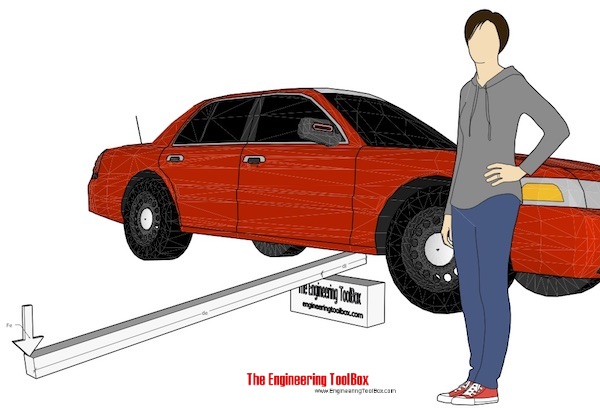An improvised car lift can be made with a piece of lumber as illustrated in the figure above. With distance load to fulcrum 0.2 m, distance effort force to fulcrum 2 m and a load on the lever - half the weight of the car 2000 kg - the effort force can be calculated

Fe = (0.5 2000 kg) (9.81 m/s2) (0.2 m) / (2 m)

= 981 N

≈ 100 kg

### Orders of Levers

#### First-order levers

• the fulcrum is positioned between the effort and the load
• the effort is smaller than the load
• the effort moves further than the load
• the lever can be considered as a force magnifier

#### Second-order Levers

• the effort and the load are positioned on the same side of the fulcrum but applied in opposite directions
• the load lies between the effort and the fulcrum
• the effort is smaller than the load
• the effort moves further than the load
• the lever can be considered as a force magnifier

#### Third-order Levers

• the effort lies between the load and the fulcrum
• the effort is greater than the load
• the load moves further than the effort
• the lever can be considered as a distance magnifier

### Example - First-Class (Order) Lever - A force (weight) of 1 pound is exerted at the end of a lever at distance 1 ft from the fulcrum

The effort force at a distance of 2 ft from the fulcrum can be calculated as

Fe = (1 lb) (1 ft) / (2 ft)

= 0.5 (lb)

The formula (1) can be modified to express required load if you know the effort, or required distance from fulcrum if load and effort forces are known and so on.

The level above where the fulcrum located between the load and effort force is often characterized as a first-class level mechanism.

A level where the load and effort force are located on the same side of the fulcrum is often characterized as a second-class level mechanism.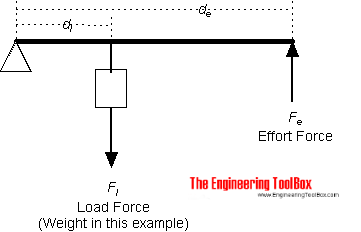### Example - Second-Class (Order) Lever

A force (weight) of 1 pound is exerted at a distance of 1 ft from the fulcrum.

The effort force at a distance of 2 ft from the fulcrum can be calculated as

Fe = (1 lb) (1 (ft) / (2 ft)

= 0.5 (lb)

### Example - Lever calculation with SI-units - weight of 1 kg mass acting 1 m from the fulcrum

The effort force at a distance of 2 m from the fulcrum can be calculated as

Fe = (1 kg) (9.81 m/s2) (1 m) / (2 m)

= 4.9 N

A lever mechanism where the input effort is higher than than the output load is often characterized as a third-class lever mechanism.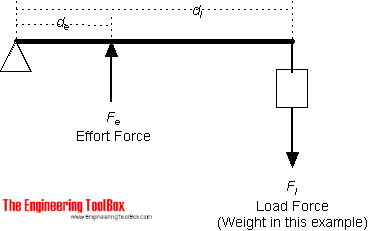### Example - Third-Class (Order) Lever

A force (weight) of 1 pound is exerted at a distance of 2 ft from the fulcrum.

The effort force at a distance of 1 ft from the fulcrum can be calculated as

Fe = Fl dl / de

= (1 lb) (2 ft) / (1 ft)

= 2 (lb)

### One or more forces acting on a lever

A lever with two acting load forces and one effort force is indicated in the sketch below: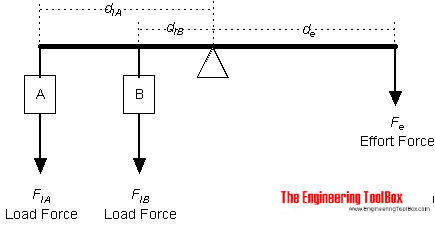The generic equation for one effort force with one or more acting load forces can be expressed as

Fe = (FlA dlA +  FlB dlB + .. + FlN dlN ) / de                                                (2)

This equation is modified for three acting loads below.

### Example - A Lever with three acting loads and one effort force

A weight A of 1 pound is exerted at a distance of 1 ft from the fulcrum. A weight B of 2 pound is exerted at a distance of 2 ft from the fulcrum, and a weight C of 3 pound is exerted at a distance of 3 ft from the fulcrum.

The effort force at a distance of 2 ft from the fulcrum can be calculated as

Fe = (FlA dlA +  FlB dlB + FlC dlC ) / de

= ((1 lb) (1 ft) + (2 lb) (2 ft) + (3 lb) (3 ft)) / (2 ft)

= 7 (lb)

## Related Topics

• ### Mechanics

Forces, acceleration, displacement, vectors, motion, momentum, energy of objects and more.
• ### Statics

Loads - forces and torque, beams and columns.

## Related Documents

• ### Cantilever Beams - Moments and Deflections

Maximum reaction forces, deflections and moments - single and uniform loads.
• ### Density vs. Specific Weight and Specific Gravity

An introduction to density, specific weight and specific gravity.
• ### Elevators - Force and Power

Required force and power to lift an elevator.
• ### Force

Newton's third law - force vs. mass and acceleration.
• ### Force Ratio

The force ratio is the load force versus the effort force.
• ### Improvised Torque Wrench

Improvise a torque wrench with a luggage scale.
• ### Mass vs. Weight

Mass vs. weight - the Gravity Force.
• ### Screw Jack - Effort Force vs. Load

Screw jacks and effort forces.

• ### Toggle Joint

A toggle joint mechanism can be used to multiply force.
• ### Torque or Moment of Force - Online Converter

Torque or moment - the tendency of a force to rotate an object.

## Engineering ToolBox - SketchUp Extension - Online 3D modeling!

Add standard and customized parametric components - like flange beams, lumbers, piping, stairs and more - to your Sketchup model with the Engineering ToolBox - SketchUp Extension - enabled for use with older versions of the amazing SketchUp Make and the newer "up to date" SketchUp Pro . Add the Engineering ToolBox extension to your SketchUp Make/Pro from the Extension Warehouse !

We don't collect information from our users. More about

## Citation

• The Engineering ToolBox (2008). Levers. [online] Available at: https://www.engineeringtoolbox.com/levers-d_1304.html [Accessed Day Month Year].

Modify the access date according your visit.

9.19.12